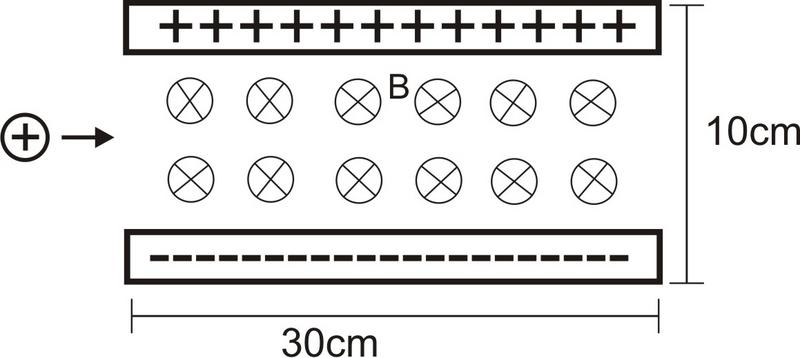# Problem with magnetic field

aldaris565

## Homework Statement

A proton travles with a velocity 8x10^5 m/s E=10^6 V/m and B= 1.5 T. Draw the path of the proton and what is speed of the proton when it comes out?

Here an image:## Answers and Replies

JaredJames
As per PF rules, you need to show your own attempt before we can help you.

Please don't double post. Use this one, not the one in General Physics.

aldaris565
Yeah ok, i'm newbie in this forum... that's what i did :

[PLAIN]http://img222.imageshack.us/img222/3104/imagengh.jpg [Broken]

But i don't know what else to do.. please help me =)

Last edited by a moderator:
JaredJames
OK, so you have the method, but where's your working?

aldaris565
Well just replace with the values given...I'm stuck i don't know if that's correct or not... I decided do it with all variables and then replace them...

I guess i sould integrate the speed getting the position (x,y) but i'm not sure if that's correct...

I need help.. I HAVE to resolve this problem...

I need ideas you don't have to resolve all the problem.. just give me ideas!!

Mentor
Well just replace with the values given...I'm stuck i don't know if that's correct or not... I decided do it with all variables and then replace them...

I guess i sould integrate the speed getting the position (x,y) but i'm not sure if that's correct...

I need help.. I HAVE to resolve this problem...

I need ideas you don't have to resolve all the problem.. just give me ideas!!

What is the shape of the path of a charged particle in a uniform magnetic field?

You correctly list the Lorentz force as the force that is acting on the proton in the magnetic field (there is no E field, right?). What does that Lorentz force do to the path of the proton? Draw the path, and plug in the numbers for the force. The force acts over a finite distance of the proton's trajectory -- what is the resulting trajectory when the proton leaves the B-field region?

aldaris565
What is the shape of the path of a charged particle in a uniform magnetic field?

You correctly list the Lorentz force as the force that is acting on the proton in the magnetic field (there is no E field, right?). What does that Lorentz force do to the path of the proton? Draw the path, and plug in the numbers for the force. The force acts over a finite distance of the proton's trajectory -- what is the resulting trajectory when the proton leaves the B-field region?

But in the problem exist an E field... the trajectory doesn't have to be exact... i guess it's a Uniform Circular Movement.

If i'd do:

First for t=0 -> x=0 y=0.05
Then:
Finding t=? for x=0.3 y=0.05

That would be correct¿? then i replace that value of t in the formula of the speed... would i get the final speed (when it comes out) ??

Mentor
But in the problem exist an E field... the trajectory doesn't have to be exact... i guess it's a Uniform Circular Movement.

If i'd do:

First for t=0 -> x=0 y=0.05
Then:
Finding t=? for x=0.3 y=0.05

That would be correct¿? then i replace that value of t in the formula of the speed... would i get the final speed (when it comes out) ??

Ah yes, I missed that there is an E field too. So you have to use the full Lorentz force in the region between the plates, and account for the deflecting forces from the E and B fields.

If it were only the B-field, then yes, the particle would trace a circular path. Given the B-field direction shown, would the proton be deflected up or down by that B-field?

But you do need to add in the contribution of the E field as well. Which way will the E-field try to deflect the proton?

Since both the E and B fields are constant in the region between the plates, you should be able to just add their contributions. However, the bending path will make the proton stay between the plates a little longer than if it travelled in a straight line, so there may be an integration involved...

Mentor
Maybe try solving separately for the E-field case and the B-field case, to see about what you can expect for the combined case, before doing any complicated integrals...

aldaris565
The integrals are no so complicated... but in another forum someone told me that the proton never leaves and the path i guess it's not a straight line, because qE is diferent that q(vxB) [q(vxB) and qE are in opossite directions but they aren't equals to eliminate them...]

Mentor
The integrals are no so complicated... but in another forum someone told me that the proton never leaves and the path i guess it's not a straight line, because qE is diferent that q(vxB) [q(vxB) and qE are in opossite directions but they aren't equals to eliminate them...]

What radius do you get for a B-field only version of this problem?